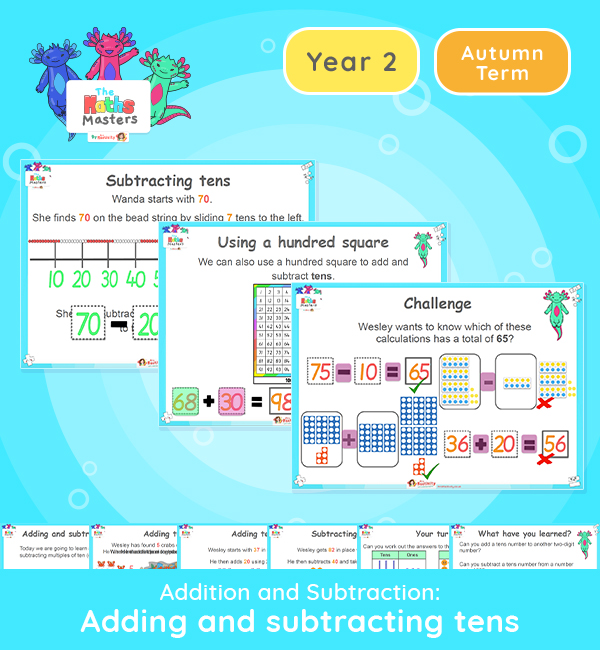# Year 2 | Add and Subtract Tens PresentationAligned with the maths mastery approach, this Year 2 | Add and Subtract Tens Presentation is fully editable, and is designed for the Year 2 maths curriculum covering the following maths objectives for the autumn term:

This lesson focuses on counting adding and subtracting tens to a given number within 100.

Small Steps: Add and subtract 10s

NC Links: Add and subtract numbers using concrete objects, pictorial representations and mentally including: a two-digit number and tens.

Year 1 Conceptual Prerequisites: Know that a multiple of 10 is made up from a number of tens.

2AS-3 Add and subtract within 100 by applying related one-digit addition and subtraction facts: add and subtract only tens to/from a two-digit number.

Future applications: Add and subtract using mental and formal written methods.

TAF Statements:          Working towards: Partition a two-digit number into tens and ones and demonstrate an understanding of place value, though they may use structured resources to support them.

Greater depth – Using reasoning about numbers and relationships to solve more complex problems and explain their thinking.

Aligned with the order of teaching of the White Rose Maths scheme of work, use this to help your class get to grips with each mathematical concept. This lesson presentation also includes varied fluency activities, problem solving, and mathematical discussion questions too.

Explore our other  year 2 addition and subtraction resources.

## Recently Viewed